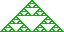Convex Hull Algorithm
Finding the minimal convex hull containing a set of 2D points is a standard problem. The standard approach is a somewhat clunky one:  "Eddy/Floyd" elimination followed by an angular sweep sort followed by a  Graham Scan.  This gives O(N log(N)) performance but is tiresome and fiddly to code and vulnerable to pathological data.

The following "divide and conquer" algorithm - though  worst case O(N2) is far more likely to be O(N log(N)) and outperforms Graham in most cases. It has the considerable advantages of computational simplicity and robustness.

It is possible to implement this algorithm by means of a single call to a general recursive primitive but as a special case serves for all but the uppermost level of the recursion we implement the simpler case recursively and code the first level explicitly.

Note that in the following a  set refers to an unordered collection of objects while a  list is an  ordered collection of obects. fset denotes the empty set while flist denotes the empty list

The basic primitive LeftHull accepts two points a and b and a  set S of points known to lie to the "left" of the line ab and to project to points between a and b. It returns a  list of points defining the remainder of the boundary of the covex hull containing a,b and S (from b to a exclusive).
We can define LeftHull as follows:

LeftHull(a,b,Sab)
if S=fset then return flist
 Set nab = æ ay-by ö è bx-ax ø

Find c Î Sab that maximises (c-a).nab giving a value > 0. If no such c exists then discard S and return flist.
 Set ncb = æ cy-by ö è bx-cx ø

Create a new point set Scb = fset.
For each pÎSab if (p-b).ncb > 0 move p from Sab to Scb.
 Set nac = æ ay-cy ö è cx-ax ø

Create a new point set Sac = fset.
For each pÎSab if (p-c).nac > 0 move p from Sab to Sac.
Discard all points remaining in Sab. (This elimiantes all points within the triangle abc from subsequent consideration.)
Return list obtained by concatenating LeftHull(c,b,Scb) + {c} + LeftHull(a,c,Sac)
End LeftHull     _message(hello 2)

The top level of the recursion is given simply a set of points S and proceeds as follows:
Find two distinct points a and b Î S known to lie on the minimal convex hull containing S. The obvious choice for a,b are the points having minimal and maximal y coordinates.
 Set nab = æ ay-by ö è bx-ax ø

Create empty sets Sab and Sba.
For each p Î S:
if (p-a).nab > 0 move p from S to Sab.
if (p-a).nab < 0 move p from S to Sba.
Discard all points remaining in S.
The unlooped edge list of the minimal convex hull of S is then given by concatenating {b} + LeftHull(b,a,Sba) + {a} +  LeftHull(a,b,Sab).

Note that if a and b are chosen by minimal and maximal y then the two "halves" of solution correspond to the left and right hand boundaries of the hull.

This method generalises for three or higher dimensional convex hulls in the obvious manner. In 3D, the recursive primitive accepts three points and a set of points known to lie to one side of the plane defined by them and to project into that plane to points within the triangle. It finds that point furthest from the plane and calls itself for three distinct subsets of the inherited set while discarding all those points within the constructed tetrahedron.

Glossary   Contents   Author
Copyright (c) Ian C G Bell 1998
Web Source: www.iancgbell.clara.net/maths or www.bigfoot.com/~iancgbell/maths
18 Nov 2006.